# MCQ in DC Circuits Part 6 | REE Board Exam

(Last Updated On: November 13, 2020)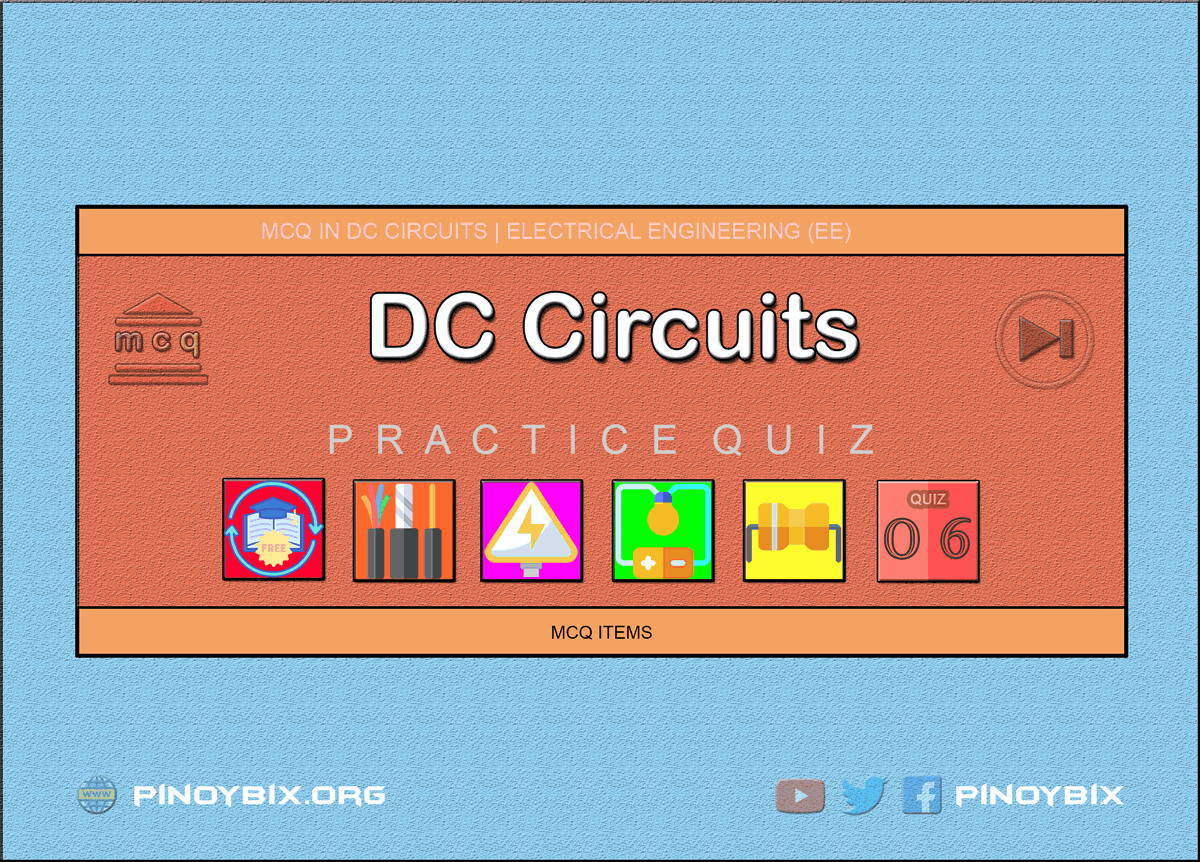This is the Multiple Choice Questions Part 6 of the Series in DC Circuits as one of the Electrical Engineering topic. In Preparation for the REE Board Exam make sure to expose yourself and familiarize in each and every questions compiled here taken from various sources including but not limited to past Board Exam Questions in Electrical Engineering field, Electrical Engineering Books, Journals and other Electrical Engineering References.

#### Continue Practice Exam Test Questions Part 6 of the Series

OHM’S LAW AND ELECTRIC CIRCUITS

Choose the letter of the best answer in each questions.

251.  Which of the following statement is TRUE both for a series and parallel d.c circuit?

D.  elements have individual currents

Solution:

252.  A 100-W, 110-V and a 50-W lamp are connected in series across a 220-V dc source. If the resistances of the two lamps are assumed to remain constant, the voltage across the 100-W lamp is ____ volt.

A.  110

B.  73.3

C.  146.7

D.  220

Solution:

253.  In  the  parallel  circuit  of  Fig.1.3,  the  value  of  V0  is _____ volt.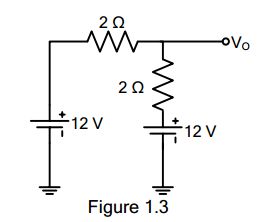A.  12

B.  24

C.  0

D.  -12

Solution:

254.  In  the  series  circuit  of  Fig  1.4,  the  value  of  V0  is ____ volt.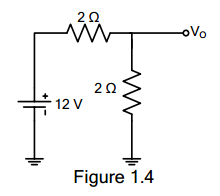A.  12

B.  -12

C.  0

D.  6

Solution:

255.  In Fig 1.5, there is a drop of 20 V on each resistor. The potential of point A would be ____ volt.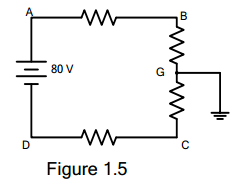A.  +80

B.  -40

C.  +40

D.  -80

Solution:

256.  From the voltmeter reading of Fig. 1.6, is it obvious that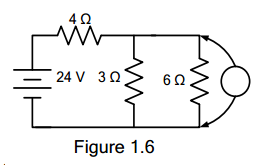A.  the 3 Ω resistor is short circuited

B.  the 6 Ω resistor is short circuited

C.  nothing is wrong with the circuit

D.  the 3 Ω resistor is open-circuited

Solution:

257.  With  reference  to  Fig  1.7,  which  of  the  following statement is true?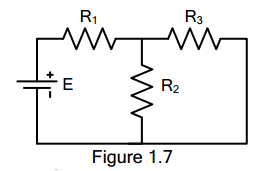A.  E and R1 form a series circuit

B.  R1 is in series with R3

C.  R1 is in series with R2

D.  there is no series circuit

Solution:

258.  Which  of  the  following  statements  is  correct concerning the Fig. 1.8?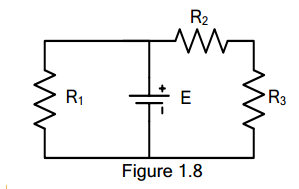A.  R2 and R3 form a series of path

B.  E is in series with R1

C.  R1 is in parallel in R3

D.  R1, R2 and R3 form a series of circuit

Solution:

259.  What is the equivalent resistance in ohms between points  A  and  B  of  Fig.  1.9?  All  resistances  are  in ohms.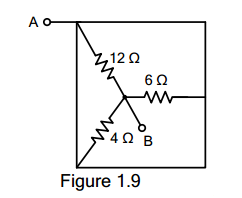A.  12

B.  14.4

C.  22

D.  2

Solution:

260.  What  do  you  call  a  resistor  that  does  not  obey Ohm’s Law?

A.  Potentiometer

B.  Carbon-Film Resistor

C.  Wire-Wound Type

D.  Non-linear Resistor

Solution:

261.  A  100  W,  110  V  and  50  W,  110  V  lamps  are connected in series across a 220 V DC source. If the  resistances  of  the  two  lamps  are  assumed  to remain constant, the voltage across the 100 W lamp is _____ volt?

A.  110 V

B.  73.3 V

C.  146.7 V

D.  220 V

Solution:

262.  A  potential  divider  of  resistance  of  50  ohms  is connected  across  a  100  V  DC  source.  A  load resistance of 10 ohms is connected across a tap in the potential divider and the negative terminal of the source.  If  a  current  of  4  A  flows  towards  the  load, what is the current supplied by the source?

A.  5.32 A

B.  5.05 A

C.  5.21 A

D.  5.48 A

Solution:

263.  Two  resistors  A  and  B  made  of  different  materials have temperature coefficients of resistance at 20°C of  0.004  and  0.006  respectively.  When  connected across a voltage source  at 20°C, they draw current equally. What  percentage  of  the  total  current  at 100°C does resistor A carry?

A.  47.14%

B.  52.86%

C.  61.34%

D.  38.66%

Solution:

264.  A conductor has a resistance of 7 ohms at 0°C. At 20°C,  the  resistance  has  become  7.5  ohms. Calculate  the  temperature  coefficient  of  the conductor at 20°C.

A.  1/300 /°C

B.  1/400 /°C

C.  1/500 /°C

D.  1/600 /°C

Solution:

265.  Which of the following is a non-linear element?

A.    diode

B.    heater coil

C.  transistor

D.  electric arc with unlike electrode

Solution:

ELECTRICAL AND HEAT ENERGY

266.  EE Board Exam April 1992

An electric kettle was marked 500 W, 230 V found to  take  15  minutes  to  bring  1  kilogram  of  water  at 15°C to boiling point. Determine the heat efficiency of the kettle.

A.  79.1%

B.  75.3%

C.  72.4%

D.  74.8%

Solution:

267.  REE Board Exam October 1997

A process equipment contains 100 gallons of water at  25°C.  It  is  required  to  bring  it  to  boiling  in  10 minutes. The heat loss is estimated to be 5%. What is the kW rating of the heater?

A.  125 kW

B.  252 kW

C.  50.5 kW

D.  207 kW

Solution:

268.  EE October 1989

A total of 0.8 kg of water at 20°C is placed in a 1-kW electric kettle. How long a time in minute is needed to raise the temperature of the water to 100°C?

A.  4.46 min

B.  5.32 min

C.  5.34 min

D.  4,.56 min

Solution:

269.  REE October 1998

How  many  calories  does  an  electric  heater  of  100 watts generate per second?

A.  10

B.  1000

C.  23.88

D.  42.25

Solution:

270.  REE Board Exam October 1997

The  electric  energy  required  to  raise  the temperature of water in a pool is 1000 kWh. If the heat  losses  are  25%,  the  heating  energy  required will be ______.

A.  1111 kWh

B.  1266 kWh

C.  1750 kWh

D.  1333 kWh

Solution:

271.  EE Board Exam April 1992

An  electric  heater  carries  12  A  at  110  V,  is submerged  in  22.5  lbs  of  water  for  30  minutes. What will be the final temperature of the water if its initial temperature is 35°F?

A.  135.43°F

B.  125.42°F

C.  133.56°F

D.  128.33°F

Solution:

272.  EE Board Exam October 1990

In  an  electric heater  the  inlet temperature  is  15°C. Water  is  flowing  at  the  rate  of  300  grams  per minute. The voltmeter measuring voltage across the heating  element  reads  120  volts  and  an  ammeter measuring  current  taken  reads  10  amperes. When steady  state  is  finally  reached,  what  is  the  final reading of the outlet thermometer?

A.  57.6°C

B.  68.4°C

C.  72.6°C

D.  42.6°C

Solution:

273.  EE Board Exam October 1991

Four  cubic  meters  of  water  is  to  be  heated  by means  of  four  1.5  kW,  230-V  immersion  heating elements. Assuming the efficiency of the heater as 90%,  determine  the  time  required  in  boiling  the water if the initial temperature is 20°C and if all four elements are connected in parallel.

A.  71 hrs

B.  63 hrs

C.  69 hrs

D.  66 hrs

Solution:

274.  EE Board Exam October 1991

Four  cubic  meters  of  water  is  to  be  heated  by means  of  four  1.5  kW,  230-V  immersion  heating elements. Assuming the efficiency of the heater as 90%,  determine  the  time  required  in  boiling  the water  if  the  initial  temperature  is  20°C  and  if  the elements  are  connected  two  in  series  in  parallel with two in series.

A.  275.6 hrs

B.  295.3 hrs

C.  252.2 hrs

D.  264.4 hrs

Solution:

275.  REE Board Exam September 2001

How many joules per second are then in 10 watts?

A.  10

B.  5

C.  20

D.  24.5

Solution:

276.  REE Board Exam September 2001

1013 ergs/sec is equal to how many kilowatts?

A.  1,000

B.  250

C.  100

D.  10

Solution:

277.  REE Board Exam September 2000

What is 1 kW-hr in BTU?

A.  4,186

B.  3,413

C.  746

D.  1,000

Solution:

278.  REE Board Exam October 1998

What  is  the  work  in  ergs  needed  to  raise  a  10  g weight 100 m up?

A.  4.9 x 105

B.  9.8 x 107

C.  98 x 107

D.  1.96 x 107

Solution:

279.  REE Board Exam October 1999

The  quantity  of  heat  required  to  raise  the temperature of water by 1°C.

A.  energy

B.  specific heat

C.  calorie

D.  BTU

Solution:

280.  REE Board Exam October 1999

When  heat  is  transferred  into  any  other  form  of energy or when other forms of energy are converted into  heat,  the  total  amount  of  energy  is  constant. This is known as

A.  First law of thermodynamics

B.  Boyle’s law

C.  Specific heat

D.  Isothermal expansion

Solution:

281.  REE Board Exam September 2002

What is 1012 ergs/sec in kW?

A.  100 kW

B.  1,000 kW

C.  10 kW

D.  10,000 kW

Solution:

282.  REE Board Exam October 2000

An electric heater is  used to heat up 600 grams of water. It takes 14 minutes to raise the temperature of water by 40°C. If the supply voltage is 220 volts, what  is  the  power  rating  of  the  heater  neglecting heat losses?

A.  180 W

B.  120 W

C.  200 W

D.  60 W

Solution:

283.  REE Board Exam April 2001

A  100  liter  of  water  is  heated  from  20°C  to  40°C. How many kWHR of electricity is needed assuming no heat loss?

A.  4.2

B.  2.3

C.  5.6

D.  3.7

Solution:

284.  REE Board Exam April 2002

Ten (10) kW is equal to ____ gram-cal/sec.

A.  156

B.  436

C.  2,388

D.  425

Solution:

285.  ECE Board Exam November 1995

Two heaters A and B are in parallel across supply voltage  V.   Heater  A  produces  500  kcal  in  20 minutes  and  B  produces  1000  kcal  in  10  minutes. The  resistance  of  A  is  10  ohms.   What  is  the resistance of B, if the same heaters are connected in series voltage V?

A.  4.5 ohms

B.  2.5 ohms

C.  4.5 ohms

D.  0.14 ohm

Solution:

286.  In the SI system of units, the unit of force is

A.  kg-wt

B.  Newton

C.  Joule

D.  N-m

Solution:

287.  The basic unit of electric charge is

A.  ampere-hour

B.  watt-hour

C.  coulomb

Solution:

288.  The SI unit of energy is

A.  Joule

B.  kWh

C.  kcal

D.  m-kg

Solution:

289.  The SI unit of energy is

A.  Joule

B.  kWh

C.  kcal

D.  m-kg

Solution:

290.  Two heating elements, each of 230-V, 3.5 kW rating are first joined in parallel and then in series to heat same  amount  of  water  through  the  same  range  of temperature. The ratio of the time taken in the two cases would be

A.  1:2

B.  2:1

C.  1:4

D.  4:1

Solution:

291.  If  a  220  V  heater  is  used  on  110  V  supply,  heat produced by it will be ____ as much

A.  one-half

B.  twice

C.  one-fourth

D.  four times

Solution:

292.  For  a  given  line  voltage,  four  heating  coils  will produce maximum heat when connected

A.  all in parallel

B.  all in series

C.  with two parallel pairs in series

D.  one pair in parallel with the other two in series

Solution:

293.  The  electric  energy  required  to  raise  the temperature  of  a  given  amount  of  water  is  1000 kWh.  If  heat  losses  are  25%,  the  total  heating energy required is ____ kWh.

A.  1500

B.  1250

C.  1333

D.  1000

Solution:

294.  One kWh of energy equals nearly

A.  1000 W

B.  860 kcal

C.  4186 J

D.  735.5 W

Solution:

295.  A force of 10,000 N accelerates a body to velocity 0.1 km/s. The power developed is ____ kW.

A.  1,000,000

B.  36,000

C.  3600

D.  1000

Solution:

296.  A 100 W light bulb burns on an average of 10 hours a  day  for  one  week.  The  weekly  consumption  of energy will be ____ unit/s.

A.  7

B.  70

C.  0.7

D.  0.07

Solution:

297.  Two heaters, rated at 1000 W, 250 volts each, are connected  in  series  across  a  250  volt,  50  Hz  A.C. mains.  The  total  power  drawn  from  the  supply would be ____ watt.

A.  1000

B.  500

C.  250

D.  2000

Solution:

298.  One watt is equal to ____.

A.  4.19 cal/sec

B.  778 BTU/sec

C.  107 ergs/sec

D.  10-7 ergs/sec

Solution:

299.  The current in an electric lamp is 5 amperes. What quantity of electricity flows toward the filament in 6 minutes?

A.  30 C

B.  3600 C

C.  72 C

D.  1800 C

Solution:

300.  An  electric heater is rated at 120 volts, 1000 watts and  is  used  to  boil  water.  Calculate  the  time  in minutes to raise the temperature of 1 liter of water from  15°C  to  boiling.  The  heater  has  an  over-all efficiency of 92%.

A.  6.4 minutes

B.  5.4 minutes

C.  4.4 minutes

D.  3.4 minutes

Solution:

#### Questions and Answers in DC Circuits

Following is the list of practice exam test questions in this brand new series:

MCQ in DC Circuits
PART 1: MCQ from Number 1 – 50                Answer key: included
PART 2: MCQ from Number 51 – 100            Answer key: included
PART 3: MCQ from Number 101 – 150           Answer key: included
PART 4: MCQ from Number 151 – 200           Answer key: included
PART 5: MCQ from Number 201 – 250           Answer key: included
PART 6: MCQ from Number 251 – 300           Answer key: included
PART 7: MCQ from Number 301 – 350           Answer key: included
PART 8: MCQ from Number 351 – 400            Answer key: included
PART 9: MCQ from Number 401 – 450            Answer key: included
PART 10: MCQ from Number 451 – 500          Answer key: included

PinoyBIX Engineering. © 2014-2020 All Rights Reserved | How to Donate? |